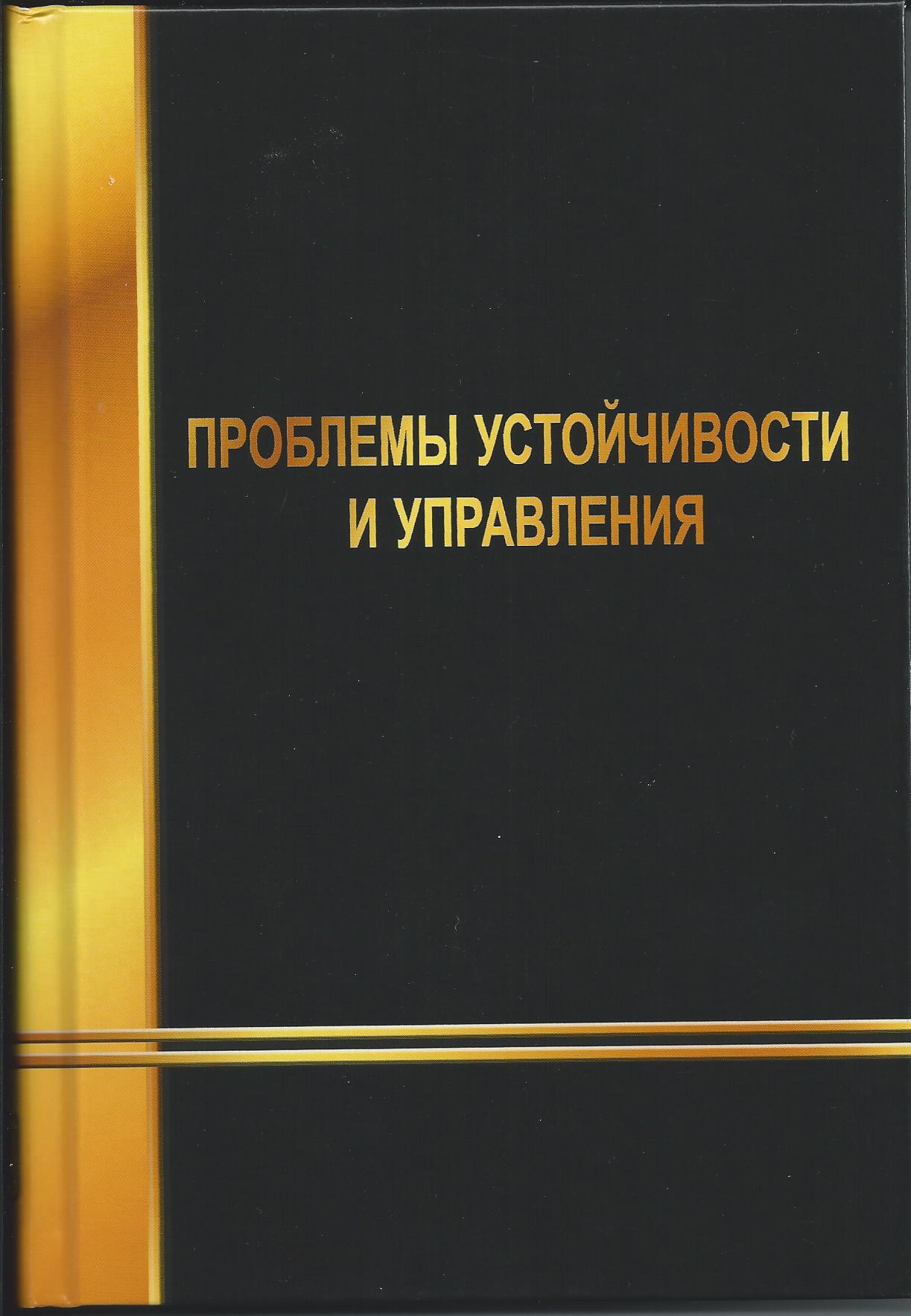• A
• A
• A
• ABC
• ABC
• ABC
• А
• А
• А
• А
• А
Regular version of the site

## Оптимальное управление нелинейным объектом, линеаризуемым обратной связью по состоянию

С. 104-117.

Mathematical models of nonlinear systems of a certain class allow them represented as linear systems with nonlinear state feedback. In other words, let make the appropriate coordinate transformation of the original dynamic model. Such a transformation, using Lyapunov functions, a number of studies used to determine the parameters of regulators to ensure the asymptotic stability properties of the nonlinear system, ie guaranteeing bounded trajectories emanating from the initial states of the system. For linear systems, there is a powerful and convenient mathematical apparatus allows the synthesis of optimal controls, but this unit is not applicable or partially applicable for nonlinear systems. Unlike prior work in this paper for nonlinear systems linearizable feedback as in the synthesis of optimal control problems with quadratic performance applied the method based on the use of the Riccati equation with parameters depending on the state.

### In bookМ.: Физматлит, 2013.